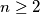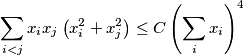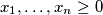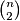### IMO Shortlist 1999 problem A1

Kvaliteta:
Avg: 0,0
Težina:
Avg: 6,0
Let$n \geq 2$ be a fixed integer. Find the least constant$C$ such the inequalityholds for any$x_{1}, \ldots ,x_{n} \geq 0$ (the sum on the left consists of$\binom{n}{2}$ summands). For this constant$C$, characterize the instances of equality.
Izvor: Međunarodna matematička olimpijada, shortlist 1999